# SAT - Section 6 Question 01

##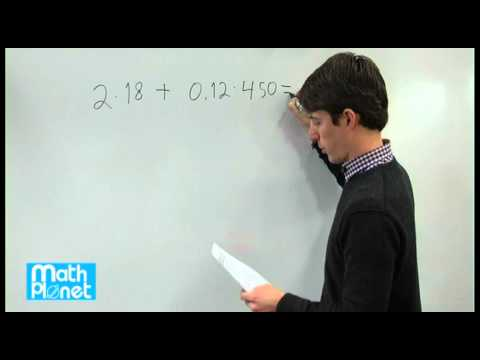By MathPlanetVideos# ACT - Question 01

##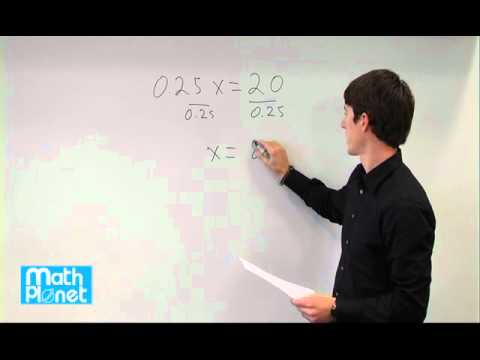By MathPlanetVideos# SAT - Section 6 Question 05

##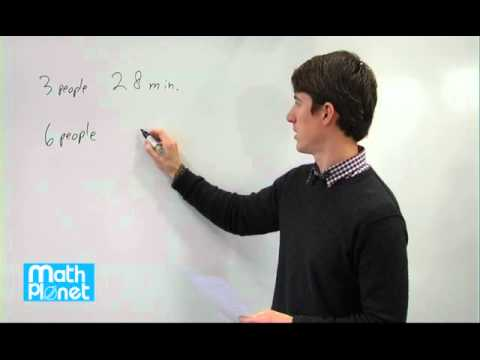By MathPlanetVideos# Application problems with equation in one variable

##By Khan Academy

Application problems with equation in one variable. All Khan Academy content is available for free at www.khanacademy.org# One-step equation intuition

##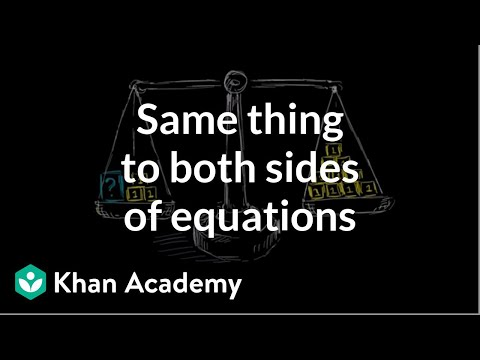By Khan Academy

The example of a scale where we try to achieve balance helps to explain why we do the same thing to both sides of an equation.# One-step equation intuition

##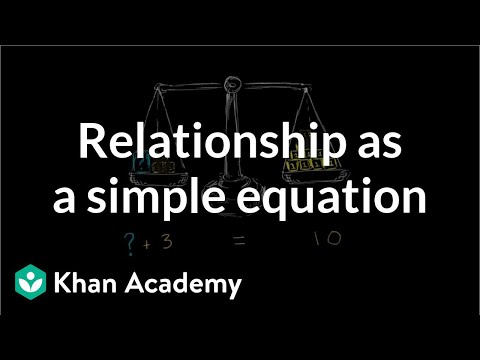By Khan Academy

Equations are about relationships (no, not girlfriends and boyfriends!) between the two sides of the equation. Let's again use a scale example to help us understand.# One-step equation intuition

##By Khan Academy

To find the value of a variable, you sometimes have to get it on one side of the equation alone. To do that, you'll need to do something to BOTH sides of the equation. Watch. We'll explain why we do this.# Two-step equations

##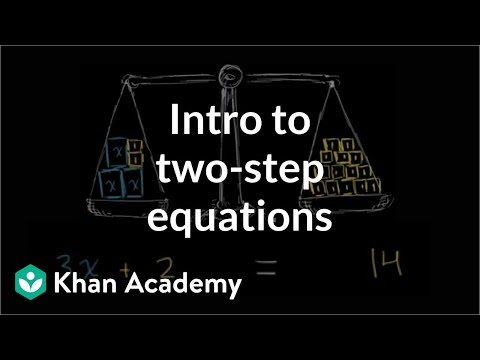By Khan Academy

Here's how we solve a two step equation. It begins with the concept of equality: what we do to one side of the equation must be done to the other.# Two-step equations

##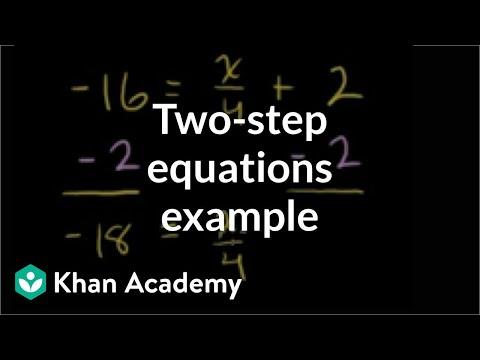By Khan Academy

Solve the equation by isolating the term with numerator x.# Two-step equations

##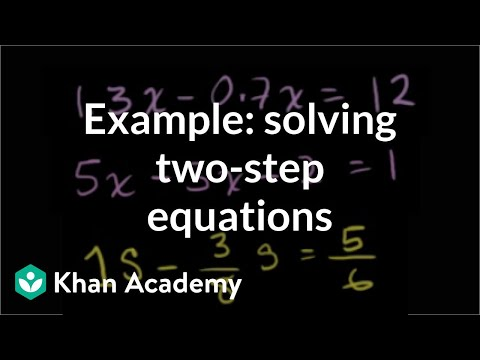By Khan Academy

Let's practice some two step equations, some of which require merging terms and using the distributive property.# Two-step equations

##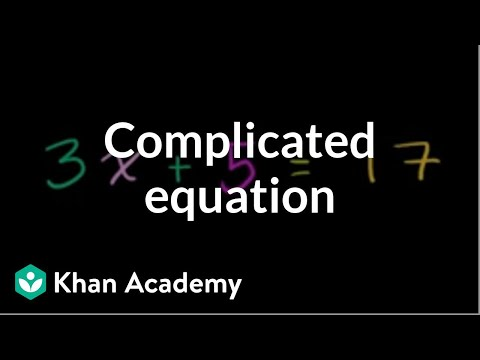By Khan Academy

This example demonstrates how we solve an equation expressed such: ax + b = c. It's a little more complicated than previous examples, but you can do it!# Linear equation word problems

##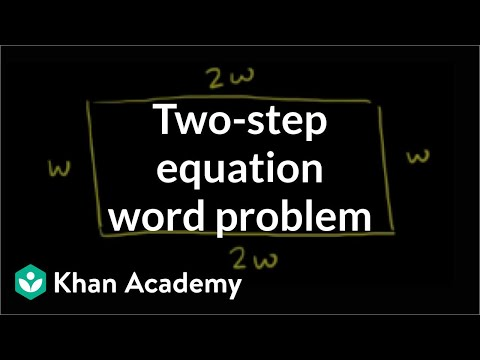By Khan Academy

Here's a nifty word problem in which we find the dimensions of a garden given only the perimeter. Let's create an equation to solve!# Linear equation word problems

##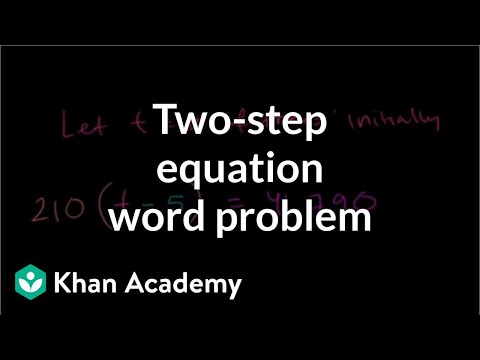By Khan Academy

Learn how to solve a word problem by writing an equation to model the situation. In this video, we use the linear equation 210(t-5) = 41,790.# Find the mistake in solving one-step equations

##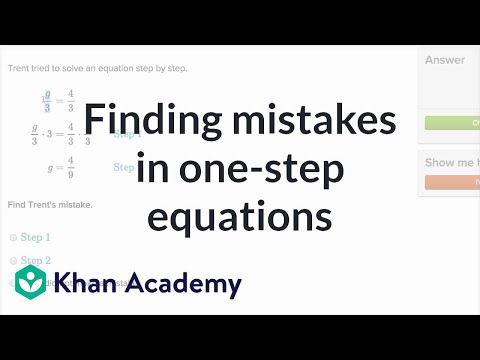By Khan Academy

Learn how to spot mistakes in someone else's work as they attempt to solve one-step equations.# 7.ee.4a

##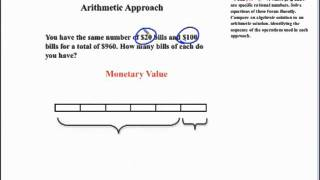By SEDL

This mathematics video is from the Common Core State Standards Video Series at http://secc.sedl.org/common_core_videos/# Multi-step rational number word problems

##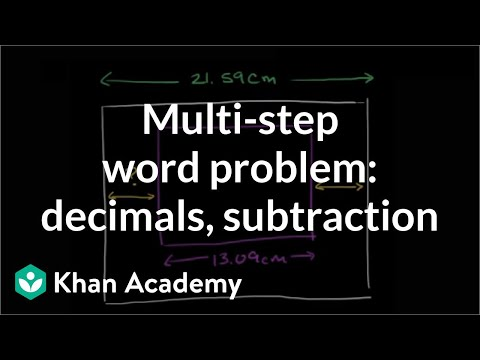By Khan Academy

First visualize this word problem then use subtraction and multiplication of decimals and fractions to get at the answer.# Multi-step rational number word problems

##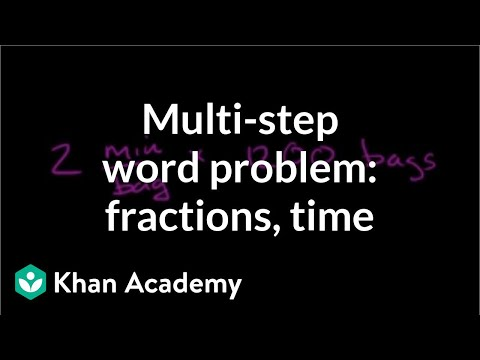By Khan Academy

Remember units of measurement? Convert minutes into hours and put your knowledge of fractions to work in this word problem.# Multi-step rational number word problems

##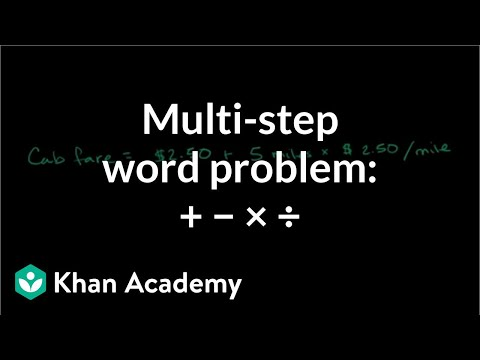By Khan Academy

Budgeting money is one of the most valuable math skills you'll learn. Practice with us as we figure out how much money is left after a cab fare.# Two-step equations with decimals and fractions

##By Khan Academy

Learn how to solve equations that involve decimals and fractions. The equations shown in this video are called two-step equations because they each take two steps to solve.# Multi-step Word Problems with Negative Numbers

##By shaunteaches

Watch this video to view two examples of multistep word problems that involve negative values.